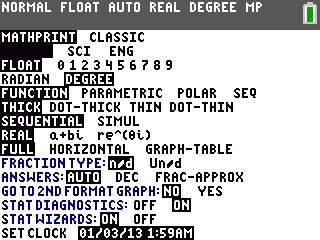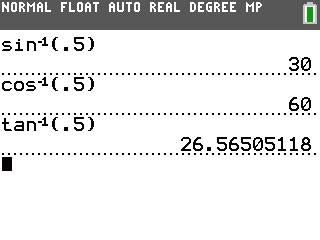# Knowledge Base

## Solution 34422: Calculating Inverse Sine (Arcsine), Cosine (Arccosine), and Tangent (Arctangent) Using the TI-84 Plus C Silver Edition Graphing Calculator.

### How do I calculate inverse sine (arcsine), cosine (arccosine), and tangent (arctangent) using TI-84 Plus C Silver Edition Graphing Calculator?

To calculate inverse sine (arcsine), cosine (arccosine), and tangent (arctangent) using the TI-83 family, TI-84 Plus family, and TI-Nspire handheld in TI-84 Plus mode, follow the steps below.

NOTE: For these examples, the handheld's angle mode setting will need to be changed to DEGREE mode.

• Press the [MODE] key.
• Scroll down to "RADIAN", scroll right to highight "DEGREE" and press [ENTER].Example: Calculate the inverse sine (arcsine) of .5.

Press [2nd] [SIN], input .5, then press [ ) ] [ENTER].

Example: Calculate the inverse cosine (arccosine) of .5.

Press [2nd] [COS], input .5, then press [ ) ] [ENTER].

Example: Calculate the inverse tangent (arctangent) of .5.

Press [2nd] [SIN], input .5, then press [ ) ] [ENTER].Please see the TI-84 Plus C Silver Edition guidebook for additional information.